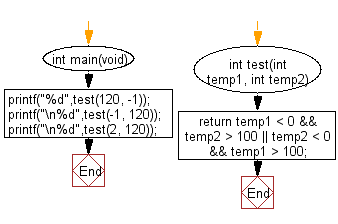﻿ C Program: Check one temperature < 0 and another > 100# C Exercises: Check whether a given temperatures is less than 0 and the other is greater than 100

## C-programming basic algorithm: Exercise-6 with Solution

Write a C program to check whether a given temperatures is less than 0 and the other is greater than 100.

C Code:

``````#include <stdio.h>
#include <stdlib.h>
int main(void){
printf("%d",test(120, -1));
printf("\n%d",test(-1, 120));
printf("\n%d",test(2, 120));
}
int test(int temp1, int temp2)
{
return temp1 < 0 && temp2 > 100 || temp2 < 0 && temp1 > 100;
}
``````

Sample Output:

```1
1
0
```

Pictorial Presentation:Flowchart:C Programming Code Editor:

What is the difficulty level of this exercise?

Test your Programming skills with w3resource's quiz.

﻿

## C Programming: Tips of the Day

Why doesn't a+++++b work?

printf("%d",a+++++b); is interpreted as (a++)++ + b according to the Maximal Munch Rule!.

++ (postfix) doesn't evaluate to an lvalue but it requires its operand to be an lvalue.

! 6.4/4 says the next preprocessing token is the longest sequence of characters that could constitute a preprocessing token"

Ref : https://bit.ly/3fdldUT